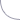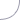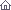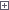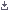Electro-Hobby Wednesday, 21.10.2020, 14:01 Circuits Welcome Guest | RSS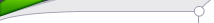Search

 StatisticsTotal online: 1 Guests: 1 Users: 0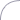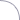Home » Tests and Measurement » Making a digital capacitance meter using microcontroller
 11:00 Making a digital capacitance meter using microcontroller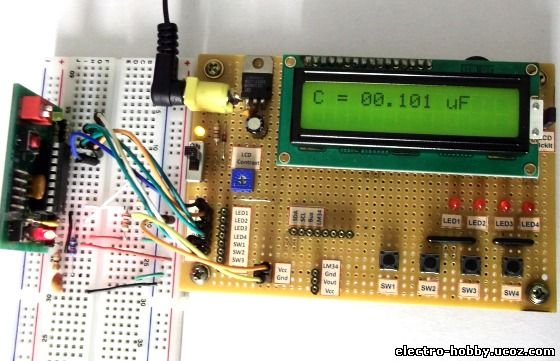Capacitors are one of the most common passive electrical components that are extensively used in all kinds of electronic circuits. In this project, we will discuss a technique of building a digital capacitance meter using a PIC microcontroller. This project can measure capacitance values from 1 nF to 99 μF, with a resolution of 1 nF. The technique is based on measuring the time elapsed when a capacitor is charged to a known voltage through a series resistor. The microcontroller used in this project is PIC16F628A.TheoryThis capacitance meter is based on the principle of charging a capacitor through a series resistor. In a series RC circuit, as shown in the figure below, the voltage across the capacitor increases exponentially as it charges. Lets assume that initially the capacitor was fully discharged. When Vin is applied across the RC circuit, the capacitor starts charging and consequently, the voltage (Vc) across it increases from 0 towards Vin in an exponential way, as shown in the right side of the figure. The equation provided in the figure describes how the voltage across the capacitor changes with time. If we know the time that is required for the capacitor to charge up to a known voltage then we can solve this equation for C, knowing the value of R.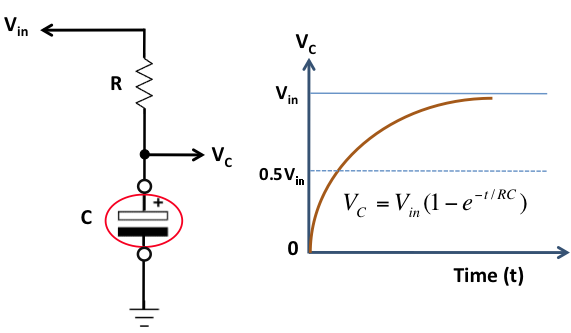Capacitor voltage increases exponentially with timeThe idea of measuring the time elapsed when the capacitor is charged from 0 to a known voltage can be implemented with any microcontroller. Here, we are using the PIC16F628A microcontroller, which has two built-in analog comparators. In this project, we are using the Analog Comparator 2 and TIMER2 modules to determine the time required by the capacitor to charge from 0V to 0.5Vin. The positive and negative inputs of the Analog Comparator 2 are externally accessible through RA2 and RA1 pins of PIC16F628A, respectively. In the figure shown below, two 2.2K resistors creates a voltage divider that sets the positive input (RA2) of the comparator to half of the voltage applied to RA0 pin. The negative input (RA1) of the comparator goes to the positive end of the capacitor through a 330Ω resistor. The resistor is used to discharge the capacitor prior to its measurement by setting RA1 low. When a voltage is applied to the RA0 pin, the capacitor (Cx), initially fully discharged, is charged through a 22K resistor. When the RA0 pin is just set high (say around 5V), the output of the comparator is high as the positive input of the comparator is at higher voltage (about 2.5V) than the negative input, which is close to 0V as the capacitor is fully discharged. Now the capacitor starts charging through the series resistor (22K) and when the voltage across it exceeds half of the voltage at RA0 pin, the comparator output is flipped to low. The comparator interrupt flag (CMIF) is set whenever there is a change in the output value of the comparator. The Timer2 module is used to compute the time elapsed between when RA0 is set high and the comparator output goes low. This is the time required by the capacitor to charge from 0V to half of the supply voltage.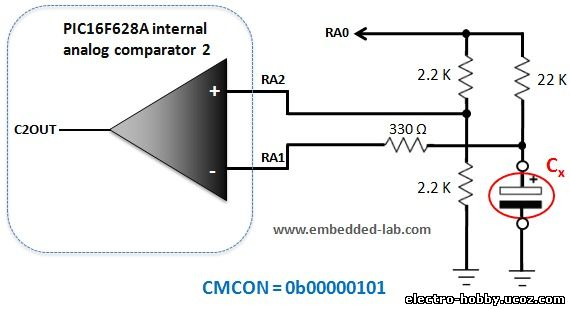By knowing the value of the charging resistor (in this case it is 22K) and the charging time (from Timer2), we can now solve the capacitor equation, mentioned above, to compute C. Here’s the mathematics involved in the process. For simplicity, the Timer2 is initialized with value 104 so that it overflows in 256-104=152 clock cycles (the number 152 comes from the math shown below). If we use 4.0 MHz external clock source, this is equivalent to 152 μsec. By doing so, the calculations are much simplified as described below. The final equation suggests that for the given arrangement, the measured capacitance (in nF) is simply 10 multiplied by the number of times the Timer2 overflows, starting from 104 every time. This gives a resolution of 10nF, which can be further improved to 1nF by considering the value of Timer2 itself at the instant when the comparator output switches to low and the Timer2 module is stopped.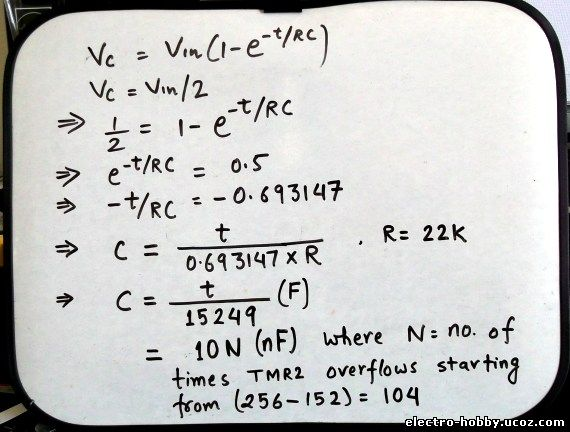The complete circuit setup for this project is given below. The pins RA0-RA2 goes to the voltage divider and capacitor charging circuit described earlier. The measurement begins when theStart push button is pressed. The measured capacitance is displayed on a standard character LCD. The +5V power supply is derived from a 9V battery using a LM7805 regulator IC.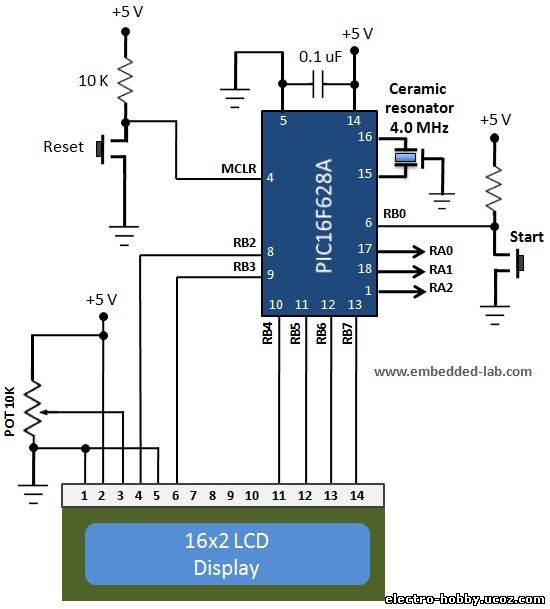SoftwareThe firmware for the PIC16F628A microcontroller is written in C and compiled with mikroC Pro for PIC compiler. The maximum value of measurable capacitance is set to 99.99 uF. The program displays "Out of Range” message while measuring capacitance value higher than this. Normally, the RA0 pin is set to low so that the capacitor gets discharged through the 22K resistor before measurement. When the Start button is pressed, the RA1 pin is set to low for 2 sec which expedites the discharging process as the capacitor discharges much quicker through the 330 Ω resistor, as compared to 22K. Next, the Timer2 is initialized and the corresponding interrupt is enabled. RA1 and RA2 are configured as analog comparator inputs. Then RA0 is set to high and Timer2 is turned on. As soon as the comparator output (CMCON.C2OUT) flips from high to low, the Timer2 module is stopped. The time required by the capacitor to charge from 0V to half of the voltage across the RC circuit is computed from the number of times the Timer2 has overflown and the final value of the Timer2 register itself. This timing information is used to estimate the capacitance value using the math described in the theory section.```/* Project: Capacitance meter Description : CapMeter based on RC time constant MCU: PIC16F28A Oscillator: HS, 4.0000 MHz external Written by: Rajendra Bhatt (www.embedded-lab.com) */ // LCD module connections sbit LCD_RS at RB2_bit; sbit LCD_EN at RB3_bit; sbit LCD_D4 at RB4_bit; sbit LCD_D5 at RB5_bit; sbit LCD_D6 at RB6_bit; sbit LCD_D7 at RB7_bit; sbit LCD_RS_Direction at TRISB2_bit; sbit LCD_EN_Direction at TRISB3_bit; sbit LCD_D4_Direction at TRISB4_bit; sbit LCD_D5_Direction at TRISB5_bit; sbit LCD_D6_Direction at TRISB6_bit; sbit LCD_D7_Direction at TRISB7_bit; sbit Va at RA0_bit; sbit Switch at RB0_bit; char message1[] = "Capacitance"; char message2[] = "Meter"; unsigned int T_Value, Num; unsigned short i, j, TimerValue, OverRange = 0; char Capacitance[] = "00.000 uF"; void interrupt(){ if(PIR1.TMR2IF){ TMR2 = TimerValue; Num ++; if(Num > 9999) OverRange = 1; // Range is 99.99 uF PIR1.TMR2IF =0; // Clear TMR0 interrupt flag } } void Display_Cap(unsigned int n){ Capacitance = n/10000 + 48; Capacitance = (n/1000)%10 + 48; Capacitance = (n/100)%10 + 48; Capacitance = (n/10)%10 + 48; Capacitance = (T_Value*10)/153 + 48; Lcd_Cmd(_Lcd_Clear); Lcd_Out(1, 1, "C = "); Lcd_Out(1, 5, Capacitance); } void reset(){ TRISA = 0b00000100; CMCON = 7; RA1_bit = 0; Delay_ms(2000); TRISA = 0b00000110; CMCON = 5; } void main(){ char cap_size; TRISB = 0b00000001; PORTB = 0; TRISA = 0b00000110; OPTION_REG.T0CS = 0; INTCON.GIE = 1; //Enable global interrupt INTCON.PEIE = 1; //Enable peripheral interrupt // Configure Timer2 module PIE1.TMR2IE = 1; // Enable Timer2 interrupt T2CON = 0; // Prescaler 1:1, and Timer2 is off initially PIR1.TMR2IF =0; // Clear int bit // Configure Comparator module CMCON = 5; // Independent comparator between RA1 (-) and RA2(+) Lcd_Init(); Lcd_Cmd(_Lcd_Clear); Lcd_Cmd(_LCD_CURSOR_OFF); Lcd_Out(1, 1, message1); Lcd_Out(2, 1, message2); delay_ms(2000); Lcd_Cmd(_Lcd_Clear); Lcd_Out(1, 1, "C = "); Lcd_Out(1, 5, Capacitance); Va = 0; TimerValue = 108; // 104 + 4 additional clock cycles delay on branching to ISR while(1){ if(!Switch) { Num = 0; OverRange =0; Lcd_Cmd(_Lcd_Clear); Lcd_Out(1, 1, "Testing."); Lcd_Out(2, 1, "..."); TMR2 = TimerValue; // Initialize Timer2 Va = 1; //apply voltage T2CON.TMR2ON = 1; // start timer while(CMCON.C2OUT) { if(OverRange) break; } T2CON.TMR2ON = 0; // stop timer T_Value = TMR2 - TimerValue; // T_Value is used for improved resolution Va = 0; //--------------------------------- if(!OverRange){ Display_Cap(Num*10); } else{ OverRange = 0; Lcd_Cmd(_Lcd_Clear); Lcd_Out(1, 1, "Out of Range!"); } reset(); } } } Download complete source and HEX files OutputA variety of capacitance values are tested with this capacitance meter and the results are found pretty consistent with their rated values. Here are some snapshots showing the meter in action.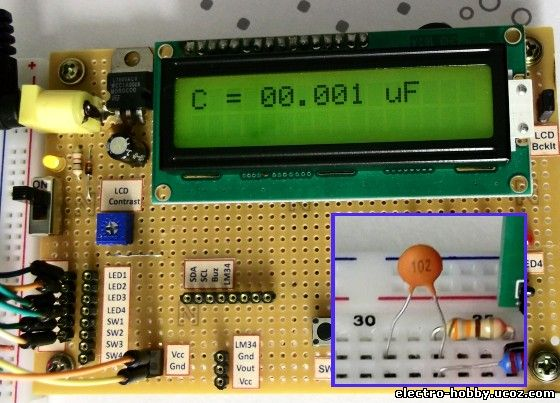SummaryThe charging phenomenon of a capacitor through a series resistor was discussed and used to construct a very basic capacitance meter. The PIC16F628A microcontroller was used to control the charging/discharging process of the capacitor. With the help of a built-in analog comparator and a timer module, the PIC microcontroller computed the time required by the capacitor to build a known voltage across it when charged through a given series resistance. Using all these information, the microcontroller computed the capacitance. The measured output was found to be reasonably consistent with the rated value when tested with a wide range of capacitance values.Note: All capacitors, but specifically high voltage capacitors, must be first properly discharged prior to measurement to avoid any damage to the capacitance meter circuit. ``` Category: Tests and Measurement | Views: 2818 |